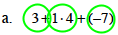### Home > MC1 > Chapter 3 > Lesson 3.3.1 > Problem3-65

3-65.

Circle terms and simplify each of the following expressions.

1. $3+1·4+(−7)$

Refer back to problem 2-132 if you need help getting started with this problem. Try doing the work on your own before checking the solution.

Begin by circling each term.Simplify the expression.

$3+4+(−7)$

$7+(−7)=0$

$0$

1. $50+3(−10)+2(−20)$

Following the same steps as in part (a), try solving this problem before checking the answer.

$−20$

1. $4+(−50)·2$

Follow the same steps as in part (a). Make sure you are circling the terms, so that simplifying is easier.

1. $(4+(−170))(5+(−4))$

In this problem, make sure to add within the parentheses before multiplying for the final product.